# How to Find a Missing Angle in a Triangle

How to Find a Missing Angle in a Triangle• The sum of the three angles in a triangle add to 180 degrees.
• 60° + 60° + 60° = 180°
• 30° + 110° + 40° = 180°
• 40° + 50° + 90° = 180°
• To find a missing angle in a triangle, subtract the two known angles from 180°.

To find a missing angle in a triangle, subtract the two known angles from 180°.• We have a triangle with 60°, 100° and one missing angle.
• The three angles will add up to 180° in total.
• Adding the 2 known angles: 60° + 100° = 160°
• We subtract this from 180° to find the missing angle.
• 180° – 160° = 20°.
• The missing angle is 20°.Supporting Lessons

#### Finding a Missing Angle in a Triangle# How to Find a Missing Angle of a Triangle

## How to Find a Missing Angle in a Triangle

To find a missing angle in a triangle, subtract the two known angles from 180°. It is sometimes easier to add the two known angles together first and then subtract this sum from 180°.

Here is an example of finding a missing angle in a triangle.

The triangle has 2 known angles, 60° and 100°.The first step is to add the two known angles together.

60° + 100° = 160°We use the fact that angles in a triangle add to 180° to find the missing angle.

160° plus the missing angle must equal 180°.

We can simply think, “What do we add to 160° to make 180°?”

160° + 20° = 180°Alternatively, 180° – 160° = 20°

The missing angle in this triangle is 20°.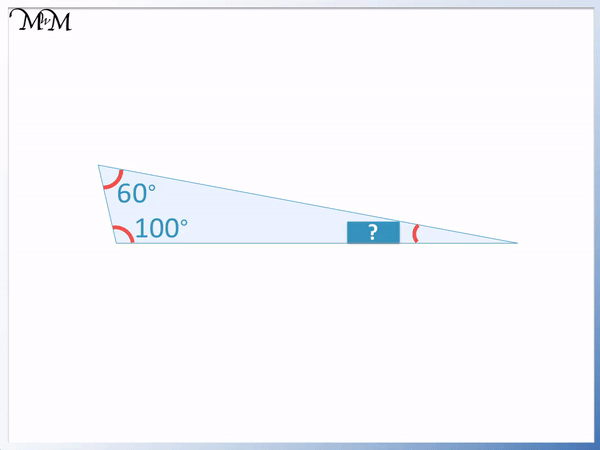We can find the missing angle by simply subtracting the known angles from 180°.

180° – 100° = 80° and then 80° – 60° = 20°.

Here is another example of finding a missing angle in a triangle.

To find the missing angle in a triangle use the following steps:

1. Add the two known angles together.
2. Subtract this result from 180°The two known angles are 94° and 40°.

The first step is to add 94° and 40°. 94° + 40° = 134°.

The next step is to subtract 134° from 180°. 180° – 134° = 46°.

The missing angle is 46°.

## Finding a Missing Acute Angle in a Right-Angled Triangle

To find the missing acute angle in a right-angled triangle, simply subtract the other acute angle from 90°.

We will first work out the missing angle by using the fact that all angles in a triangle add to 180°.

In the example below, we have a right-angled triangle with 38° and 90°. The square in the corner of the angle tells us that this angle is 90° or a right angle.We can add the two known angles together.

90° + 38° = 128°So, we think, “What do we add to 128° to make 180°?”

Or we can subtract 128° from 180°.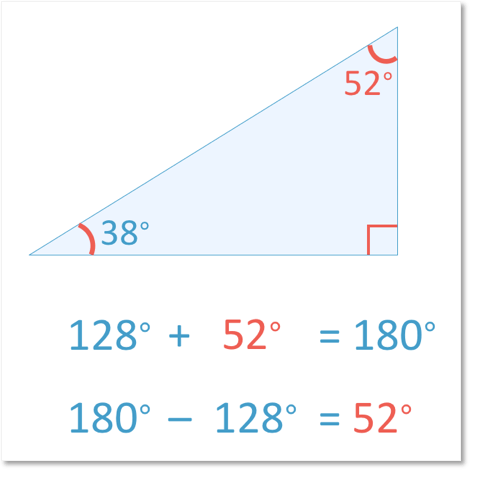180° – 128° = 52°

The missing angle in the triangle must therefore be 52°.We can see that the right angle is 90°. We can subtract the 90° from 180° to begin with.

180° – 90° = 90° and so, the two acute angles must add up to equal 90°. Therefore 38° plus the unknown angle must add up to 90°.

Therefore if the triangle is right-angled, then it can be easier to find a missing acute angle by simply subtracting the other acute angle from 90°.

90° – 38° = 52° and so, the missing angle is 52°.

## Finding a Missing Angle in an Isosceles Triangle

If two angles in an isosceles triangle are unknown and are the same size, then subtract the known angle from 180° and then divide this result by 2.

Here is an example of finding two missing angles.

The one known angle is 30°. We know that the other two angles are equal in size since there are two lines marked on the triangle opposite each angle.We subtract the known angle from 180° and then divide the result by 2.

180° – 30° = 150° and 150° ÷ 2 = 75°.Both of the missing angles are equal to 75°.

## Finding the Missing Angles in an Equilateral Triangle

All of the three angles in an equilateral triangle are equal to 60°. This is because all three angles add up to 180° and the three angles are equal in size. 180° divided by 3 is 60°. Here is an equilateral triangle.

All angles in an equilateral triangle are 60°.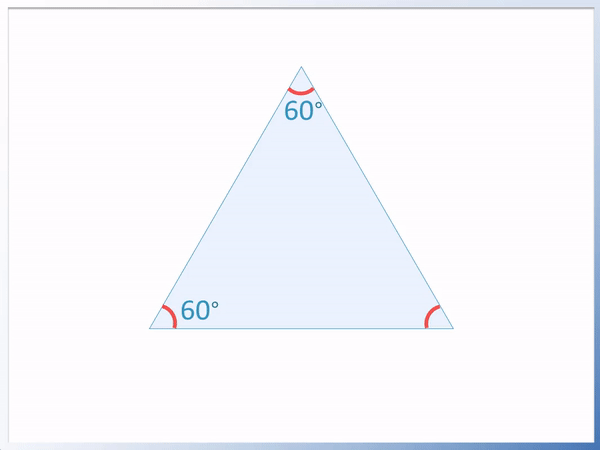An equilateral triangle can be identified by the three lines on each of its sides. These lines tell us that the three sides are all the same size and so, the three angles are also the same size.

Here is a diagram of an equilateral triangle with three lines marking each side.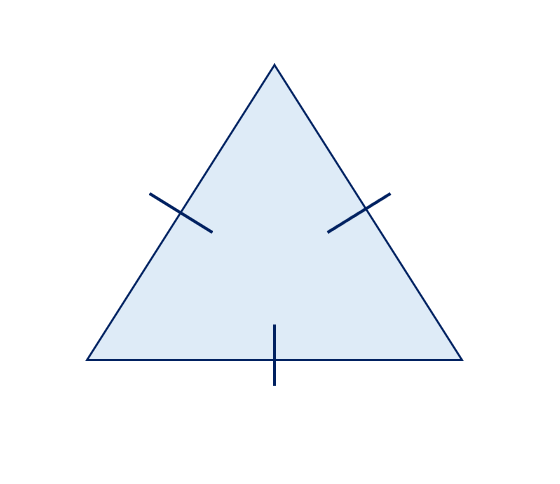Every triangle that has three sides the same length is an equilateral triangle and all of its three angles will be 60.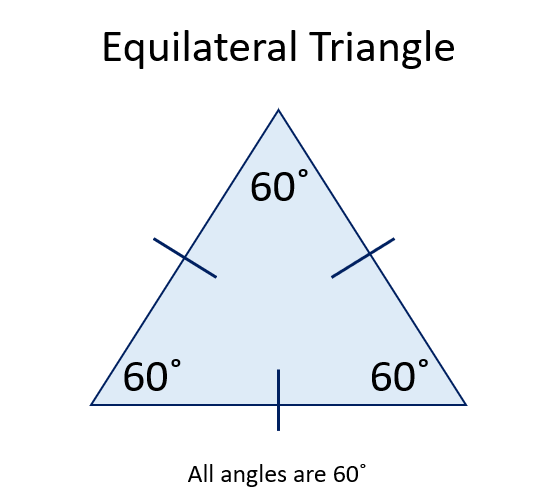## How to Show that Angles in a Triangle Add to 180°

To show that angles in a triangle add to 180°, cut off each of the three angles and place them together to form a straight line. A straight line is equal to 180° and so, angles in a triangle are also equal to 180°.

When teaching angles in a triangle, it is a useful and fun exercise to show that the angles in all triangles add to 180°. You can draw any triangle you wish and cut it out. Testing a range of different triangles can help explain that this rule works for every triangle. It is also fun for children to come up with their own example.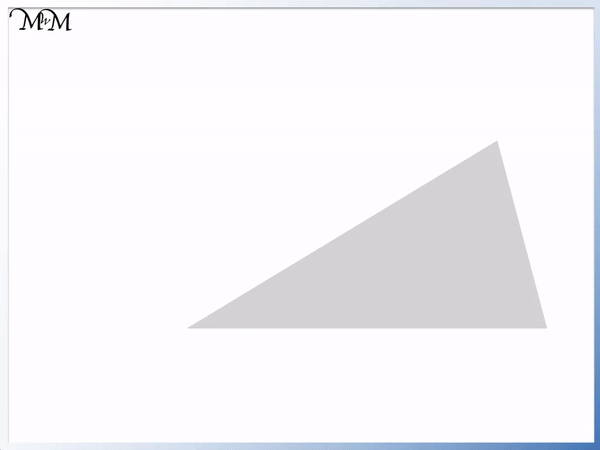Here are the steps for showing that angles in a triangle add to 180°:

1. Cut out a triangle.
2. Mark the outer angles.
3. Cut these angles off.
4. Place these angles together so that they form a straight line with no gaps.
5. Draw a straight line below them.
6.1) Cut out a triangle2) Mark the outer angles3) Cut these angles off4) Place these marked angles togetherYou should be able to place these angles onto a straight line.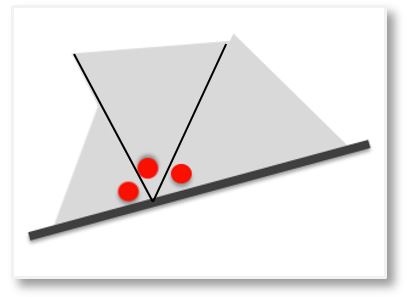You can also show that angles in a triangle add to 180° by teaching this using a protractor. Simply draw out any triangle of your choice and measure the angles with a protractor.

The angles will always add to 180°.

Here is an example where the angles add to 40°, 50° and 90°. 40° + 50° + 90° = 180°.Here is another example of a triangle with angles 30°, 110° and 40°.

Again 30° + 110° + 40° = 180°.Now try our lesson on How to Find Isosceles Triangle Angles where we learn what an isosceles triangle is and how to find missing angles in isosceles triangles.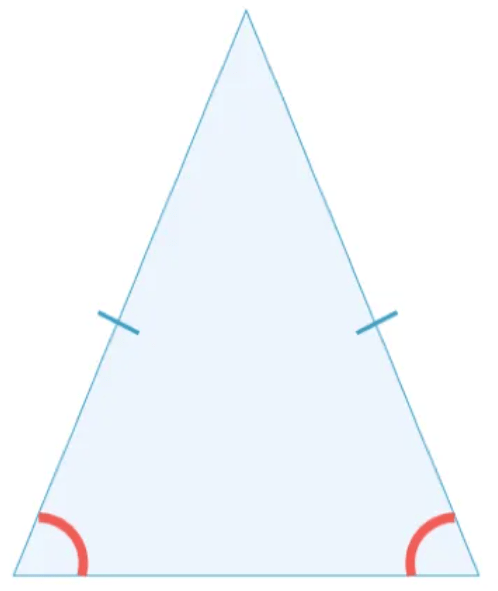error: Content is protected !!